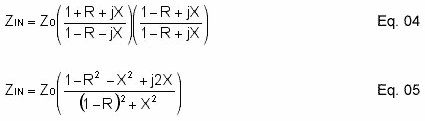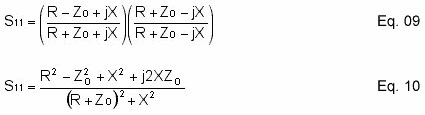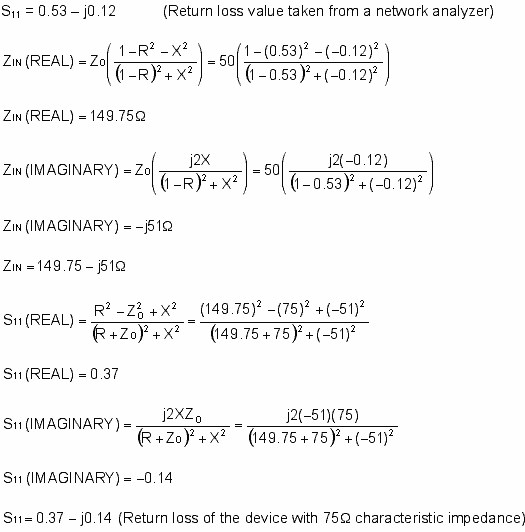# Converting S-Parameters from 50Ω to 75Ω Impedance

### Abstract

Cable television integrated circuits are usually designed to interface with 75Ω input and output impedance, while most RF test equipment is 50Ω impedance. In most cases, minimum loss pads are used to convert the input and output impedance from 75Ω to 50Ω, and visa versa, for testing purposes. Although convenient, minimum loss pads should be avoided when measuring S-parameters. One easy way to perform such measurement is by treating the input and output impedance of the cable device as 50Ω, perform the test, and use the equations and Excel spreadsheet presented in this application note to convert the 50Ω-referenced S-parameters back to 75Ω.

### Introduction

Cable television integrated circuits are usually designed to interface with 75Ω inputs and outputs, while most RF test equipment use 50Ω impedance. In most cases, minimum-loss pads are used to convert the input and output impedances from 75Ω to 50Ω, and vice-versa. Although convenient, minimum-loss pads should be avoided when measuring S-parameters.

This application note presents an easy way to perform S-parameter measurements by treating the input and output impedance of the cable device as 50Ω, performing the test, and making a mathematical conversion. We provide the equations and a handy Excel spreadsheet to convert 50Ω-referenced S-parameters back to 75Ω.

### S-Parameter to Impedance

The conversion of a 50Ω-referenced S-parameter to 75Ω begins with equation 1. Both the S-parameter and input impedance are complex numbers (R + jX), where R represents the real component, and the X represents the imaginary component. ZO is usually a real impedance. For the sake of simplicity, input return loss (S11) will be considered throughout this application note.Rearrange Eq. 1 to obtain an input impedance (ZIN) expression Eq. 2,Replace the S11 with R + jX,Multiply the denominator of Eq. 3 with its complex conjugate to separate the real and imaginary components,Eq. 6 is the real component of the input impedance,Eq. 7 is the imaginary component of the input impedance,### Impedance to S-Parameter

Once the complex input impedance is obtained, Eq. 1 is used once again to convert the impedance back to a 75Ω-referenced S-parameter.

Eq. 8 is the same expression as Eq. 1, except ZIN is replaced with R + jX,Multiply the denominator of Eq. 8 with its complex conjugate to separate the real and imaginary components,Eq. 11 is the real component of the input return loss (S11),Eq. 12 is the imaginary component of the input return loss (S11),Example